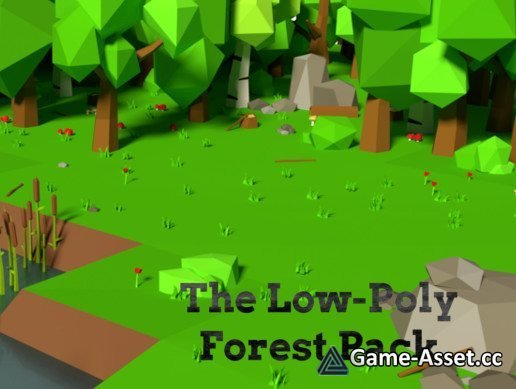SITE SEARCH

# The Low-Poly Forest Pack v1.0This pack includes 109 low-poly assets that can be used to make any low-poly forest scene you may need!

THAT'S \$0.027 PER ASSET!!!

Pack Includes:

Models = 74
Assets = 109
Textures = 1
Prefabs = 109

Exact Assets:

Oak Trees (Green Leafs) = 6
Oak Trees (Orange Leafs) = 6
Spruce Trees = 10
Birch Trees (Green leafs) = 4
Birch Trees (Orange leafs) = 4
Other Trees (Green leafs) = 9
Bushes = 6
Big Rocks = 5
Tree stumps (Oak) = 3
Tree stumps (Birch) = 3
Tree stumps (Spruce) = 3

Logs (Oak) = 3
Logs (Birch) = 3
Logs (Spruce) = 3
Sticks (Oak) = 3
Sticks (Birch) = 3
Sticks (Spruce) = 3
Small Rocks = 3
Small Rock Piles = 4

Grass = 2
Cattails = 3
Mushrooms (Red) = 5
Mushrooms (Brown) = 4
Mushrooms (Blue) = 4
Roses = 3

Asset version: 1.0
• Rating:

• RELATED NEWS